# How To Find The Area Of A Square (3 Things To Know)

The area of a square tells us how many square tiles (with a side length of 1 unit) would fit inside of the square.  It is helpful to be able to find the area of a square given information about the square.

So, how do you find the area of a square?  A square with a side length of S has an area of S*S, or S2. Side length is always given in linear units (such as feet or inches) and area is always given in square units (such as square feet or square inches). To find the side length of a square with area A, we take the square root of A, √A.

Of course, we can also find the area of a square given its perimeter, diagonal, or other information.

In this article, we’ll talk about how to find the area of a square and look at some examples.  We’ll also answer some common questions about areas of squares.

Let’s get started.

## How To Find The Area Of A Square

To find the area of a square, we need to know the side length, or find it from the information we are given.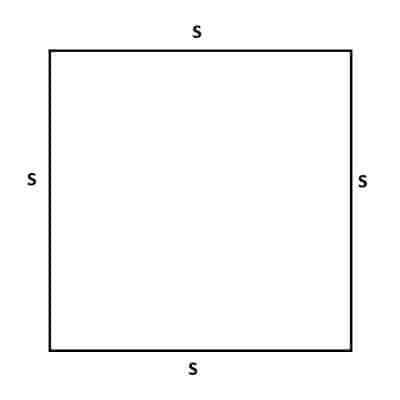If we know the side length S of a square (or if we can find it from information we are given), then we can find the area A.

Remember that a square has four sides and four 90 degree angles, with each side having the same length.  So, we only need one side length of a square and we know all the side lengths.

Let’s start off with the simplest case: finding the area of a square given its side length.

DIY Shed AsktheBuilder Shed Foundat...
DIY Shed AsktheBuilder Shed Foundation Layout

### How To Find Area Of A Square With Side Length

If we have the side length of a square, it is easy to find the area.  For a square with a side length of S, the area A is given by the formula:

• A = S2  [formula for the area of a square]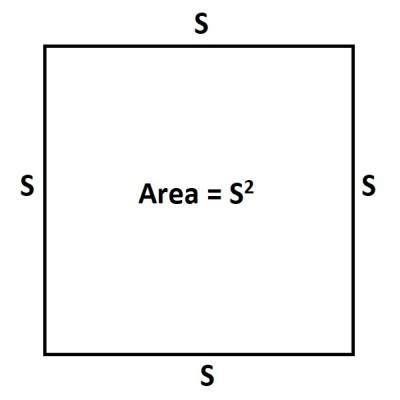A square with a side length of S has an area of S2.

Note that S (side length of the square) is given in linear units, such as:

• Feet (ft)
• Inches (in)
• Meters (m)

While A (area of the square) is given in square units, such as:

• Square Feet (ft2)
• Square Inches (in2)
• Square Meters (m2)

Be careful not to make a common mistake when converting units for area!  Remember that 1 square foot is 122 = 144 square inches (not 12 square inches!)

So, a square with an area of 4 square feet would have an area of 4*144 = 576 square inches.

Let’s look at an example of how to find the area of a square, given the side length.

#### Example: Find The Area Of A Square Given The Side Length

Let’s say we have a square with a side length of 4 feet.

Then S = 4 feet, and we can use this in the formula for the area A of a square:

• A = S2  [formula for the area of a square]
• A = 42  [units for S are feet]
• A = 16 square feet

So, the area of a square with a side length of 4 feet is 16 square feet.

We can multiply 16 by 144 to get the area in square inches: 16*144 = 2304 square inches.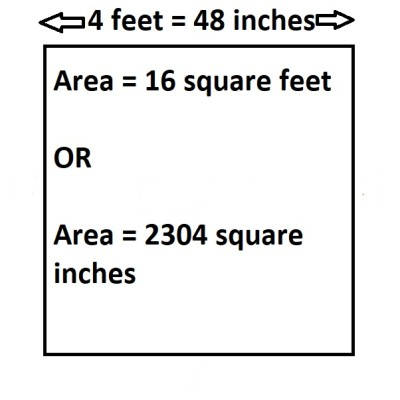A square with a side length of 4 feet (48 inches) has an area of 16 square feet (2304 square inches).

We can also convert the side length to inches and then calculate area with our formula:

• (4 feet)*(12 inches per foot) = 48 inches (side length)
• 48*48 = 2304 square inches

This is the same area that we found above.

### How To Find Area Of A Square With Perimeter

Remember that a square with a side length of S has a perimeter of P = 4S.  This comes from adding up the side lengths (there are four sides in a square, each with the same length of S):

• P = S + S + S + S = 4S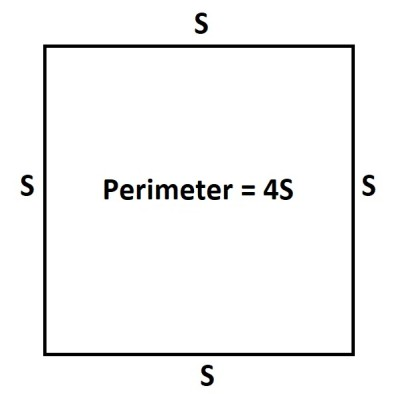A square with a side length of S has a perimeter of 4S.

We can also work backwards to find the side length S from the perimeter P.  All we need to do is divide by 4:

• P = 4S
• P/4 = S

Since we know the area A in terms of S, we can find the area in terms of the perimeter too:

• A = S2  [formula for the area of a square]
• A = (P/4)2  [solved for side length, S, in terms of perimeter, P]
• A = P2/16

The previous line is the formula for the area A of a square with perimeter P.

Note that we can also work backwards to find the perimeter P from the area A by solving:

• A = P2/16  [formula for area A in terms of perimeter P]
• 16A = P2
• 4√A = P

Let’s look at an example of how to find the area of a square given its perimeter.

#### Example: Find The Area Of A Square Given The Perimeter

Let’s say we have a square with a perimeter of 12 feet.

Then P = 12 feet, and we can use this in the formula for the area A of a square that we found above:

• A = P2/16  [formula for area A in terms of perimeter P]]
• A = 122/16  [units for P are feet]
• A = 144/16 square feet
• A = 9 square feet

So, the area of a square with a perimeter of 12 feet is 9 square feet.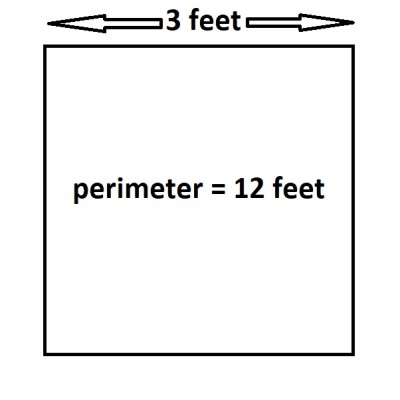A square with a perimeter of 12 feet has a side length of 3 feet, and thus an area of 9 square feet .

We can also check our work by finding the side length S.  For a square with a perimeter of 12 feet, the side length will be S = 12/4 = 3 feet.

A square with a side length of S = 3 feet will have an area of A = S2 = 32 = 9 square feet.  This matches what we found above.

### How To Find Area Of A Square With Diagonal

Remember that a square with a side length of S has a diagonal of D = S√2.  This comes from using the Pythagorean Theorem on one of the right triangles (half of the square, with the diagonal as the hypotenuse):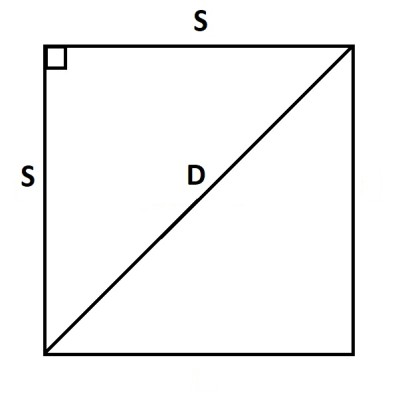A square with a diagonal D and side length S satisfies the equation D = S√2.
• a2 + b2 = c2  [Pythagorean Theorem]
• S2 + S2 = D2  [legs a and b are the side length S of the square, and c is the diagonal D]
• 2S2 = D2  [combine like terms]
• √(2S2) = D  [take square root of both sides]
• S√2 = D  [simplify]

This also means that we can solve for the side length S to find:

• S√2 = D
• S = D/√2

Since we know the area A in terms of S, we can find the area in terms of the diagonal too:

• A = S2  [formula for the area of a square]
• A = (D/√2)2  [solved for side length, S, in terms of diagonal, D]
• A = D2/2

The previous line is the formula for the area A of a square with diagonal D.

Note that we can also work backwards to find the diagonal D from the area A by solving:

• A = D2/2  [formula for area A in terms of diagonal D]
• 2A = D2
• √(2A) = D

Let’s look at an example of how to find the area of a square given its diagonal.

#### Example: Find The Area Of A Square Given The Diagonal

Let’s say we have a square with a diagonal of 10 feet.

Then D = 10 feet, and we can use this in the formula for the area A of a square that we found above:

• A = D2/2  [formula for area A in terms of diagonal D]
• A = 102/2  [units for D are feet]
• A = 100/2 square feet
• A = 50 square feet

So, the area of a square with a diagonal of 10 feet is 50 square feet.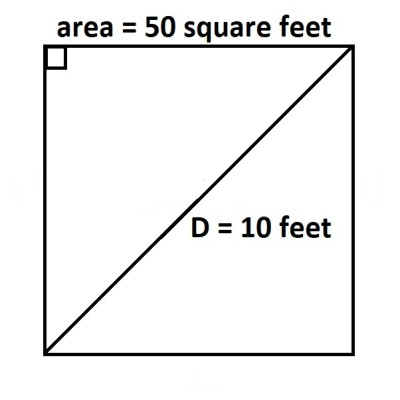A square with a diagonal 10 feet long has an area of 50 square feet.

We can also check our work by finding the side length S.  For a square with a diagonal of 10 feet, the side length will be S = D/√2 = 10/√2 = (10√2)/2 = 5√2 feet.

A square with a side length of S = 5√2 feet will have an area of A = S2 = (5√2)2 = 25*2 = 50 square feet.  This matches what we found above.

### How To Find Area Of A Square Inside A Circle

When you inscribe a square inside of a circle, you are really looking the biggest square that will just barely fit inside of the circle.  You can also think of it as the smallest possible circle that just barely contains the square.

You can see this scenario in the picture below.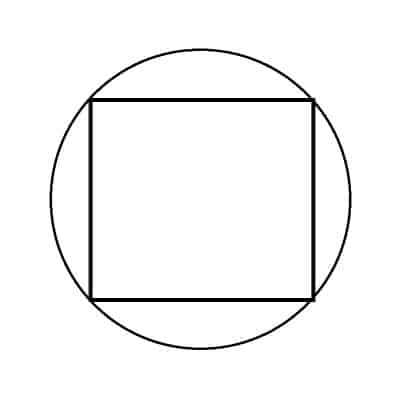Here we see the largest square that can fit inside the circle (or the smallest circle that can contain the square).

We can find the relationship between these two shapes and their dimensions as follows.

Let S be the side length of the square, and let R be the radius of the circle.

First, we will draw a diameter from the bottom left corner of the square to the top left corner.  This gives us a diagonal with length R + R = 2R (the diameter of the circle).

We will label the sides of the square “S” (as shown in the diagram below).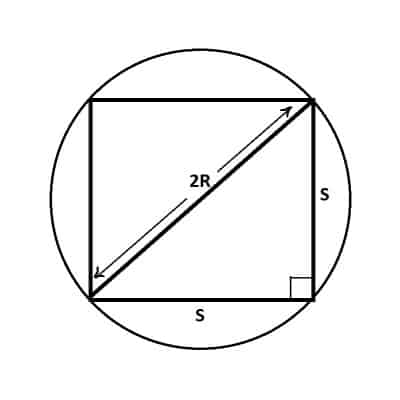The diagonal of the square is twice the radius (diameter) of the circle, or 2R. The side lengths are also labeled on the right triangle.

At this point, we have a right triangle whose two short sides (legs) have lengths of S and S, with a hypotenuse of 2R (the circle’s diameter).  Using the Pythagorean Theorem, we get:

• a2 + b2 = c2  [a and b are the side lengths, and c is the hypotenuse]
• S2 + S2 = (2R)2  [the side lengths are both S, and the hypotenuse or diagonal is 2R, the circle’s diameter]
• 2S2 = 4R2  [combine like terms]
• S2 = 2R2  [divide by 2]
• S = R√2  [take the square root of both sides]

This equation gives us the connection between the radius R of the circle and the side length S of the square.

This tells us that the side length S of the square will be about 41% larger than the radius of the circle (√2 is about 1.41 as a decimal).

The area of the square is S2 = (R√2)2 = 2R2.

Let’s look at an example.

#### Example: How To Find Area Of A Square Inside A Circle

Let’s say that we have a square inscribed inside a circle, as above.  The circle has a diameter of 12 feet.

This means that the radius (half the diameter) is 12/2 = 6 feet.  So, R = 6 feet.

We can now use the formula we found above for the area A of the square inscribed in the circle:

• A = 2R2  [area of the square inscribed in the circle of radius R]
• A = 2(62)  [R = 6 feet]
• A = 2(36) square feet
• A = 72 square feet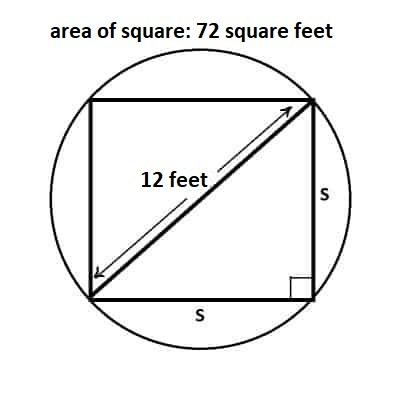A square inscribed in a circle of diameter 12 feet has an area of 72 square feet.

You can learn how to find the area of a square that contains a circle here.

### How To Find The Side Length Of A Square When Area Is Given

To find the side length of a square when the area is given, we just need to take the square root of the area.

Remember that for a square with side length S, the area is A = S2.

Taking the square root of both sides of the equation gives us √A = S.

#### Example: Find The Side Length Of A Square Given The Area

Let’s say we have a square with an area of 144 square feet.

Then A = 144, and we can find the side length S with the formula we found above:

• S = √A
• S = √144  [A = 144 square feet]
• S = 12 feetA square with an area of 144 square feet has a side length of 12 feet.

### What Does The Area Of A Square Mean?

The area of a square basically tells us how many “unit squares” fit inside of the square.  A unit square is just a square with a side length of 1 unit (the unit could be feet, inches, etc.)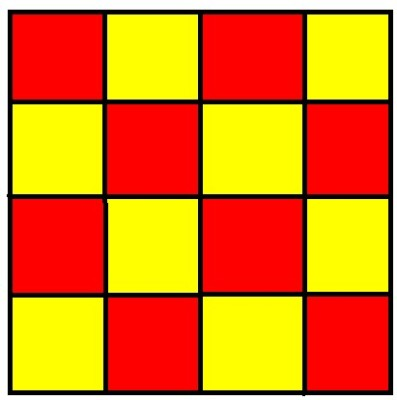A square with area 16 square feet (side length of 4 feet) would hold 16 tiles of area 1 square foot (unit square tiles).

For example, a square with a side length of 14 feet has an area of 14*14 = 196 square feet.  This square would contain 196 unit squares (squares with side lengths of 1 foot).

Another way to think about it is that a square room that is 14 feet by 14 feet would need 196 square tiles (1 foot by 1 foot) to cover the entire floor.

### Can The Perimeter & Area Of A Square Be The Same?

The perimeter and area of a square can have the same numerical value in a certain case.  However, the units are always different, since:

• Perimeter has linear units (feet, inches, meters, etc.)
• Area has square units (square feet, square inches, square meters, etc.)

Let’s use the formula for perimeter and area of a square to find out when they have the same value.

Remember that for a square with a side length of S:

• Perimeter = P = 4S
• Area = A = S2

So, the perimeter and area are the same when:

• Perimeter = Area
• 4S = S2
• 0 = S2 – 4S
• 0 = S(S – 4)
• S = 0 or S = 4

So, there are two possible cases when the perimeter and area of a square have the same value:

• S = 0 (a trivial square with no area and zero side lengths and perimeter)
• S = 4 (a square with side lengths 4 units)

So, a square with a side length of 4 feet would have a perimeter of 4*4 = 16 feet and an area of 42 = 16 square feet.

## Conclusion

Now you know how to find the area of a square.  You also know the answers to some common questions about squares and their areas.

You can learn about uses of area in real life here.

You can learn about the area of a circle here.

I hope you found this article helpful.  If so, please share it with someone who can use the information.